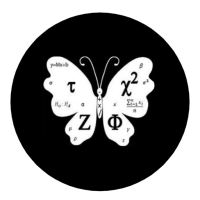## Welcome to Moore Statistics Consulting LLCOkay so you are calculating you just mastered how to construct a confidence interval for interval and ratio variables. But what about binomial variables ? How do you go about calculating the standard deviation for binomial variables? Why did the teacher not give me the standard error for a binomial problem? There must be some mistake! Actually, you have it. No really it’s there….just take the probability of success (p) and the probability of failure (q)  and use the following formula….sqrt(p*q/n) where n is your sample size. In order for you to talk about the standard deviation , you must put it in terms of the variable type. Frequency statistics is the ‘home ‘ for binomials in terms of standard deviation. Even if you are only given the number of success out of the sample, you can still obtain the frequency of success (p or #successes/sample size) and get the rest.

-Moore to follow-Amy